# LOW-DENSITY PARITY-CHECK CODED SIGNALING OVER A PARALLEL MULTICHANNEL

NOTE: This section is Under-Edit if necessary: Construction began on July 20, 2020 and was finished on July 22, 2020.

Low-Density Parity-Check (LDPC) Binary Codes: Signaling over a Parallel MultiChannel and Iterative Message-Passing Channel Decoding

```by Darrell A. Nolta
July 22, 2020 with September 23, 2020 Update
```

The AdvDCSMT1DCSS (T1) Professional (T1 Version 2) system tool now offers the capabilities to construct Gallager, Array, and Repeat-Accumulate (RA) Low-Density Parity-Check (LDPC) Binary Codes (Linear Block Codes) and to model and simulate Low-Density Parity-Check (LDPC) Coded Signaling over a Parallel MultiChannel (PMC) or MultiCarrier/MultiChannel using these LDPC Codes. In addition to these capabilities, T1 V2 supports the capability of modeling and simulating Multiple Iteration Soft Input/Soft-Decision Output (SISO) LDPC Code Channel Decoding using the Sum-Product Algorithm (SPA). The SPA is a 'symbol-by-symbol' Maximum a Posteriori Probability (MAP) Belief Propagation Algorithm. Also, T1 V2 supports the capability to model and simulate the Multiple Iteration Hard Decision Bit Flipping Algorithm Decoder. These decoding algorithms can be used in the cases for simulated LDPC Coded Signaling over a PMC with Additive White Gaussian Noise (AWGN).

This capability of T1 Professional supports decoding of LDPC Coded M-ary Signaling over a Additive White Gaussian Noise (AWGN) and Crosstalk (Xtalk) Parallel MultiChannels with AWGN; and Discrete MultiTone (DMT) Modulation MultiCarrier/MultiChannel [Orthogonal frequency-division Multiplexing (OFDM) FFT-Based] with AWGN.

A Low-Density Parity-Check Coded Parallel MultiChannel (MC) is partitioned into G parallel subchannel groups where a subchannel group consists of K parallel subchannels. The set {G * Ng} represents the possible partitions of the LDPC Code's blocklengh (N). This approach is used for the LDPC Code & Signaling over a PMC application because N can be very large and the process of Codebits to Channel Input Bits assignment can quickly become unmanageable. Note {li} is the Group's set of the Number of Channel Input Bits.

The following Parallel MultiChannel (PMC) description can refer to K-MultiChannel (K-MC) Group (G) for a LDPC Coding PMC application or K-MultiChannel for an UnCoded PMC application:

Parallel K-MultiChannel (SubChannel) Types:

Distinct or Identical (Small K); or Identical (Large K);

Bit and Power Allocation MultiChannel Parameters: Effective/Average Channel Input Bits per Subchannel Use and "Water Filling" Parameter-to-Noise Ratio VNR, Ev/No;

The MultiChannel can have a flat or Non-flat Frequency Response. The MultiChannel Linear Filter models are the same as in the Single Channel case;

For AWGN MultiChannels, the subchannels can exist as a Set of Linear Filters with Identical Impulse Responses or as a Set of Linear Filters constructed from a Single Linear Filter;

For XTalk MultiChannels, the subchannels can exist as a Set of Linear Filters with Identical Impulse Responses;

For DMT MultiChannels, the MultiChannel can exist as a Single Linear Filter;

For XTalk MultiChannels, a fraction of each Subchannel's Transmitter Info Bit Baseband SNR is propagated completely (transmitter to receiver). Each subchannel's fraction is dependent on the amount of crosstalk derived from that subchannel;

Low-Density Parity-Check (LDPC) Coded DMT Systems: combination of LDPC Codes, DMT MultiCarrier/MultiChannel Signaling and LDPC Code Channel Decoder;

LDPC Coded FFT-based DMT Discrete Time Waveform AWGN Modulation Channel Type: 1) MultiCarrier Signal transmitted over a Single Channel or 2) MultiCarrier Signal transmitted over a MC SubChannel. The Single Channel or MC SubChannel can exists as a Single Linear Filter;

For LDPC Coded DMT Signaling over a LF MC Channel, an Impulse Response-based or DMT Symbol-based Cyclic Prefix (CP) may be used [only Impulse Response-based CP can be used for the Impulse (Unit-Sample Sequence) LF MC Channel].

Note: T1 Professional supports Orthogonal Binary PSK (PI/2 BPSK) and Square 256-QAM for Low-Density Parity-Check Coded Signaling over a Parallel MultiChannel. These schemes are in addition to the other T1 supported Modulation Schemes (PSK: M = 2, 4, 8, 16, 32, 64; PAM: M = 2, 4, 8, 16, 32, 64; QAM; M = 4, 16, 64; FSK: M = 2, 4, 8, 16, 32, 64).

Next, we describe Channel Decoding of Low-Density Parity-Check Encoded Signals sent over a Parallel MultiChannel.

In summary, T1 Professional's Low-Density Parity-Check (LDPC) Code Iterative Message-Passing Channel Decoders are: Sum-Product Algorithm (Soft Decision) and Bit Flipping Algorithm (Hard Decision);

The Sum-Product Algorithm (SPA) Decoder exists as:

Model 1 (Bit Messages then Check Messages Iteration Processing) or Model 2 (Check Messages then Bit Messages Iteration Processing);

Bit Estimation Likelihood Decision Rule: MAP (Maximum A Posteriori Probability) or ML (Maximum Likelihood) for an Unequiprobable i.i.d. Binary Source (AWGN PMC); ML for Equiprobable i.i.d., All-Zero i.i.d., or Markov Binary Source [XTALK PMC or DMT PMC];

Implementation Type [for Check Message (Check Node to Bit Node Message) Calculation]: Theoretical SPA, Replace SPA Product Term by Sum, or Replace SPA Product Term by Min-Sum;

User Specified Maximum Number of SPA Decoding Iterations per Block (Imax): 3000 MAX;

The Bit Flipping Algorithm (BFA) Decoder exists as:

Bit Estimation Decision Rule: MAP (Maximum A Posteriori Probability) or ML (Maximum Likelihood) for an Unequiprobable i.i.d. Binary Source (AWGN PMC); ML for Equiprobable i.i.d., All-Zero i.i.d., or Markov Binary Source [XTALK PMC or DMT PMC];

User Specified Maximum Number of BFA Decoding Iterations per Block (Imax): 3000 MAX;

T1 Professional implements a SPA and BFA LDPC Code Decoder Figure-of-Merit:

Maximum Number of Iterations per Block (All Blocks) or Number of 'Detected Errors'; and

Average (Sample Mean) and Sample Variance of Number of Iterations per Block;

Also, T1 Professional implements M-ary Signaling Demodulation Constellation Demappers (M > 2 Search Algorithms):

Search by Bit Position and Value Partition (Soft Decision) for Low-Density Parity-Check (LDPC) Coded AWGN Parallel MultiChannel/MultiCarrier Channels (PMC) [Demodulation for LDPC Decoding Metric Calculation using Max-Log or Log-Sum Bit Metrics (User-specified)] and Constellation Demapper Decision Rule for LDPC Decoding: MAP or ML for an Unequiprobable i.i.d. Binary Source (AWGN PMC); ML for Equiprobable i.i.d., All-Zero i.i.d., or Markov Binary Source (XTALK PMC or DMT PMC).

This paper will focus on the application of T1 V2 in the study of the use of Low-Density Parity-Check Coding and Decoding to reduce the Information Bit Errors caused by AWGN in a Discrete-Time Waveform (DTW) AWGN Parallel MultiChannel and in a Discrete-Time (DT) DMT Parallel MultiCarrier Channel.

We will determine which LDPC Code (taken from the set of four possible Regular Gallager Codes) provides the best Information Bit Error Rate performance for the two chosen simulated PMC channels (AWGN & DMT).

And we will attempt to answer a very important question: Which channel (AWGN Parallel MultiChannel or DMT Parallel MultiChannel) is best for signaling over when using a LDPC Code and Soft Decision Decoding Algorithm (SPA)? The metric chosen to answer this question will be based on the minimal Information Bit Error Rate for a specific Information Bit Signal-to-Noise Ratio (Eb/N0).

Four LDPC Codes, Regular Gallager Code: [N = 126 (Rate = 0.516), 252 (Rate = 0.258), 504 (Rate = 0.254), and 504 (Rate = 0.504) and three Subchannel Groups construction (Identical 7-MC G, Distinct 5-MC G, and Distinct 6-MC G) are used.

Ideally, we want to select a Subchannel group (G & K) that would maximize the PMC Channel Input Bits (Codebits) transferred through the PMC and minimize the Information Bit Signal-to-Noise Ratio for a given Bit Error Rate.

The Subchannel Group selection is based on the number of Gray-coded Square 64-QAM Signaling subchannels per group. The Identical (I) 7-MC Group case will transmit seven 64-QAM signals in parallel. The Distinct (D) 5-MC G case will transmit three 64-QAM signals in parallel and the D 6-MC G will transmit two 64-QAM signals in parallel. Specifically, the 5-MC G Signaling Scheme will consists of {li} = {1,2,6,6,6} <=> {BPSK,QPSK, 64-QAM,64-QAM,64-QAM}. The 6-MC G Signaling Scheme will consists of {li} = {1,2,2,4,6,6} <=> {BPSK, QPSK,QPSK,16-QAM,64-QAM,64-QAM}.

Each DTW AWGN PMC subchannel transmission consists of half-cosine orthonormal baseband shaping pulse, 8 symbols per symbol period, and half-cosine matched filter demodulator front-end.

These DTW channels will possess a NonDistorting, UnRestricted Bandwidth.

The LDPC Coded FFT-based DMT DT Waveform AWGN Modulation Channel Type used is the MultiCarrier Signal transmitted over a Single Channel. Each SubChannel Group's modulation schemes are used to construct the DMT MultiCarrier Signal where the number of multicarrier frequencies (tones) is equal to G times K. The LDPC Code's G & K (derived from LDPC Code's Blocklengh N) will determine the number of Inverse Discrete Fourier Transform (IDFT) Samples of the MultiCarrier Signal. Note that for UnCoded DT DMT PMC, the K parameter will dictate the number of IDFT Samples.

Consider the SPA Decoding Algorithm Bit Error Rate (BER) or Bit Error Probability Pb performance simulation results for Regular Gallager Coded (RGC) Generator-based Encoding and Signaling that were produced by T1 that are displayed below in Figure 1, 2, 3, 4, 5, 6, and 7 plots for the AWGN PMC. In addition, consider Figure 8, 9, 10, 11, 12, 13, and 14 plots for the DMT PMC. For comparison purposes, the simulated BER results for UnCoded (UC) Gray-coded Square 64-QAM Signaling over an Identical 7-MC PMC for 10,000,032 Equal probable i.i.d. Info Bits are included in each of these figures. Figure 15, 16, 17, and 18 will provide a comparison between AWGN PMC and DMT PMC for the RGC Code N = 126 (Rate = 0.516), 252 (Rate = 0.258), 504 (Rate = 0.254), and 504 (Rate = 0.504) cases, respectively.

Each figure's BER plot displays one or more curves where a curve is constructed from the set of simulated Pb values that correspond to a set of Eb/N0 [Signal-to-Noise Ratio (SNR)] values. Thus, a BER curve is represented as {(Eb/N0, Pb)} for a number of T1 Professional simulations. Each RGC curve displays the performance behavior of a Regular Gallager Coding and SPA Iterative Decoding system example that was used for a T1 V2 simulation.

Since a PMC simulation consists of a number of Signaling Schemes (Identical or Distinct) the possible choices for the set of Signal Scheme's Eb/N0(k) values can become very large. To simplify this matter, for each Pb simulation, all the Signaling Schemes' Eb/N0(k) values are specified so that they are all equal. Thus, a plot's Eb/N0 value is defined as

Eb/N0 = Eb/N0(1) = Eb/N0(2) = … = Eb/N0(K) , for 1 through K Signaling Schemes.

Figure 1 displays the BER versus Eb/N0 for the Maximum Number of Iterations per Block (Imax) of 50 for [Number of Code Word Bits (N) = 126, column weight (j) = 3, row weight (k) = 6] Regular Gallager Coded Signaling over an AWGN PMC (I 7-MC G, D 5-MC G, D 6-MC G) for 1,000,025 Equal probable i.i.d. Information (Info) Bits.

Figure 2 displays the BER versus Eb/N0 for Imax of 50 for (N = 252, j = 3, k = 4) Regular Gallager Coded Signaling over an AWGN PMC (I 7-MC G, D 5-MC G, D 6-MC G) for 1,000,025 Equal probable i.i.d. Info Bits.

Figure 3 displays the BER versus Eb/N0 for Imax of 50 for (N = 504, j = 3, k = 4, Rate = 0.254) Regular Gallager Coded Signaling over an AWGN PMC (I 7-MC G, D 5-MC G, D 6-MC G) for 1,000,064 Equal probable i.i.d. Info Bits.

Figure 4 displays the BER versus Eb/N0 for Imax of 50 for (N = 504, j = 3, k = 6, Rate = 0.504) Regular Gallager Coded Signaling over an AWGN PMC (I 7-MC G, D 5-MC G, D 6-MC G) for 1,000,252 Equal probable i.i.d. Info Bits.

Figure 5 displays the BER versus Eb/N0 for Imax of 50 for [N = 126, 252, 504 (R = 0.254), 504 (R = 0.504)] Regular Gallager Coded Signaling over Identical 7-MC G AWGN PMC for 1,000,025, 1,000,025, 1,000,064, 1,000,252 Equal probable i.i.d. Info Bits, respectively.

Figure 6 displays the BER versus Eb/N0 for Imax of 50 for [N = 126, 252, 504 (R = 0.254), 504 (R = 0.504)] Regular Gallager Coded Signaling over Distinct 5-MC G AWGN PMC for 1,000,025, 1,000,025, 1,000,064, 1,000,252 Equal probable i.i.d. Info Bits, respectively.

Figure 7 displays the BER versus Eb/N0 for Imax of 50 for (N = 126, 252, 504 (R = 0.254), 504 (R = 0.504)]) Regular Gallager Coded Signaling over Distinct 6-MC G AWGN PMC for 1,000,025, 1,000,025, 1,000,064, 1,000,252 Equal probable i.i.d. Info Bits, respectively.

Figure 8 displays the BER versus Eb/N0 for the Maximum Number of Iterations per Block (Imax) of 50 for [Number of Code Word Bits (N) = 126, column weight (j) = 3, row weight (k) = 6] Regular Gallager Coded Signaling over a DMT PMC (I 7-MC G, D 5-MC G, D 6-MC G) for 1,000,025 Equal probable i.i.d. Information (Info) Bits.

Figure 9 displays the BER versus Eb/N0 for Imax of 50 for (N = 252, j = 3, k = 4) Regular Gallager Coded Signaling over a DMT PMC (I 7-MC G, D 5-MC G, D 6-MC G) for 1,000,025 Equal probable i.i.d. Info Bits.

Figure 10 displays the BER versus Eb/N0 for Imax of 50 for (N = 504, j = 3, k = 4, Rate = 0.254) Regular Gallager Coded Signaling over a DMT PMC (I 7-MC G, D 5-MC G, D 6-MC G) for 1,000,064 Equal probable i.i.d. Info Bits.

Figure 11 displays the BER versus Eb/N0 for Imax of 50 for (N = 504, j = 3, k = 6, Rate = 0.504) Regular Gallager Coded Signaling over an DMT PMC (I 7-MC G, D 5-MC G, D 6-MC G) for 1,000,252 Equal probable i.i.d. Info Bits.

Figure 12 displays the BER versus Eb/N0 for Imax of 50 for [N = 126, 252, 504 (R = 0.254), 504 (R = 0.504)] Regular Gallager Coded Signaling over Identical 7-MC G DMT PMC for 1,000,025, 1,000,025, 1,000,064, 1,000,252 Equal probable i.i.d. Info Bits, respectively.

Figure 13 displays the BER versus Eb/N0 for Imax of 50 for [N = 126, 252, 504 (R = 0.254), 504 (R = 0.504)] Regular Gallager Coded Signaling over Distinct 5-MC G DMT PMC for 1,000,025, 1,000,025, 1,000,064, 1,000,252 Equal probable i.i.d. Info Bits, respectively.

Figure 14 displays the BER versus Eb/N0 for Imax of 50 for (N = 126, 252, 504 (R = 0.254), 504 (R = 0.504)] ) Regular Gallager Coded Signaling over Distinct 6-MC G DMT PMC for 1,000,025, 1,000,025, 1,000,064, 1,000,252 Equal probable i.i.d. Info Bits, respectively.

Figure 15 displays the BER versus Eb/N0 for Imax of 50 for N = 126 (R = 0.518) Regular Gallager Coded Signaling over Identical 7-MC G AWGN & DMT PMC; Distinct 5-MC G AWGN & DMT PMC; Distinct 6-MC G AWGN & DMT PMC.

Figure 16 displays the BER versus Eb/N0 for Imax of 50 for N = 252 (R = 0.258) Regular Gallager Coded Signaling over Identical 7-MC G AWGN & DMT PMC; Distinct 5-MC G AWGN & DMT PMC; Distinct 6-MC G AWGN & DMT PMC.

Figure 17 displays the BER versus Eb/N0 for Imax of 50 for N = 504 (R = 0.254) Regular Gallager Coded Signaling over Identical 7-MC G AWGN & DMT PMC; Distinct 5-MC G AWGN & DMT PMC; Distinct 6-MC G AWGN & DMT PMC.

Figure 18 displays the BER versus Eb/N0 for Imax of 50 for N = 504 (R = 0.504) Regular Gallager Coded Signaling over Identical 7-MC G AWGN & DMT PMC; Distinct 5-MC G AWGN & DMT PMC; Distinct 6-MC G AWGN & DMT PMC.

One Million plus Info Bits for each Regular Gallager Coded Signaling and SPA Decoding system T1V2 simulation was chosen to allow for reasonable simulation time to generate simulated BER results that can be use to make a 1st order comparison of BER performance between j = 3 Regular Gallager Coded Signaling over a Parallel MultiChannel and SPA Decoding. Still, it must be realized that to obtain accurate BER results for long block code lengths, simulation time could take many hours or days (depending on the User's computer). Note that Dr. Gallager discusses this issue in his 1962 paper  and 1963 book .

There are a number of important conclusions that can be drawn from the below displayed simulated Iterative LDPC Code SPA Decoding BER results. It appears that T1 is correctly modeling and simulating LDPC Coded Signaling over a Parallel MultiChannel/MultiCarrier Channel with AWGN with SPA Decoding for the selected Regular Gallager Codes.

Each figure of Figure 1 through 4 clearly shows that the reduction in BER as the SNR is increased for the Regular Gallager Coded (RGC) Signaling over an Identical 7-MC Group, Distinct 5-MC Group, & Distinct 6-MC Group AWGN PMC with SPA Decoding as compared to the UnCoded Gray-Coded Square 64-QAM Signaling over an Identical 7-MC AWGN PMC.. The RGC BER curve exhibits the expected behavior as compared to the UC BER curve (i.e. a SNR region where the RGC BER is greater than the UC BER, BER is equal to each other, and, then, a SNR region where the RGC BER is less than the UC BER). Each figure's RGC BER curve exhibits a montonically decreasing curve with no fluctuations or error floor except for Figure 2 (N = 252 & D 5-MC G) and Figure 4 [N = 504 (R = 0.504) & D 5-MC G].

As expected, the best BER performance is exhibited by the Distinct 6-MC G AWGN PMC case for each selected Regular Gallager Code [N = 126, 252, 504 (R = 0.254), 504 (R = 0.504)]. But we note that the Effective (Average) Channel Input Bits (l) per SubChannel Use for the Distinct 6-MC G is 3.5 as compared to 6 for I 7-MC G and 4.2 for D 5-MC G. So if we want the best BER Performance, we have to deal with the CodeBit Rate reduction.

Next, from Figure 5, 6, and 7, we observe the BER behavior for the selected four Regular Gallager Codes for each Subchannel Group for AWGN PMC {I 7-MC G, D 5-MC G, D 6-MC G}, respectively.

From the observation of the SNR value where the BER curve begins its reduction and steepness of the initial BER reduction, we can judge the best BER performance. For I 7-MC G AWGN PMC (Figure 5), N = 504 (R = 0.254) code exhibits the best BER performance. For D 5-MC G AWGN PMC (Figure 6), N = 504 (R = 0.254) code exhibits the best BER performance. For D 6-MC G AWGN PMC (Figure 7), N = 504 (R = 0.254) code exhibits the best BER performance.

Each figure of Figure 8 through 11 clearly shows that the reduction in BER as the SNR is increased for the Regular Gallager Coded (RGC) Signaling over an Identical 7-MC Group, Distinct 5-MC Group, & Distinct 6-MC Group DMT PMC with SPA Decoding as compared to the UnCoded Gray-Coded Square 64-QAM Signaling over an Identical 7-MC DMT PMC. The RGC BER curve exhibits the expected behavior as compared to the UC BER curve (i.e. a SNR region where the RGC BER is greater than the UC BER, BER is equal to each other, and, then, a SNR region where the RGC BER is less than the UC BER). Each figure's RGC BER curve exhibits a montonically decreasing curve with no fluctuations or error floor except for Figure 9 (N = 252 & D 5-MC G) .

As expected, the best BER performance is exhibited by the Distinct 6-MC G DMT PMC case for each selected Regular Gallager Code [N = 126, 252, 504 (R = 0.254), 504 (R = 0.504)]. But we note that the Effective (Average) Channel Input Bits (l) per SubChannel Use for the Distinct 6-MC G is 3.5 as compared to 6 for I 7-MC G and 4.2 for D 5-MC G. So if we want the best BER Performance, we have to deal with the CodeBit Rate Reduction.

Next, from Figure 12, 13, and 14, we observe the BER behavior for the selected four Regular Gallager Codes for each Subchannel Group for DMT PMC {I 7-MC G, D 5-MC G, D 6-MC G}, respectively.

From the observation of the SNR value where the BER curve begins its reduction and steepness of the initial BER reduction, we can judge the best BER performance. For I 7-MC G DMT PMC (Figure 12), N = 504 (R = 0.254) code exhibits the best BER performance. For D 5-MC G DMT PMC (Figure 13), N = 504 (R = 0.254) code exhibits the best BER performance. For D 6-MC G DMT PMC (Figure 14), N = 504 (R = 0.254) code exhibits the best BER performance.

From Figure 5, 6, & 7 and Figure 12, 13, & 14, if we compare BER performance for two different Code Rates (1/4 & 1/2) for N = 504, we see that the Rate ~ 1/4 Regular Gallager Code outperforms the Rate ~ 1/2 Regular Gallager Code.

Next, we attempt to answer the question: which LDPC Coded PMC channel is best to signal over when SPA decoding is used. First, we should realize that the simulated DTW AWGN PMC with AWGN and DT DMT (OFDM FFT-Based) PMC with AWGN channels are fundamental different in structure and operation.

The DTW AWGN PMC channel consists of G number of K subchannels where each gth subchannel group operates in parallel and its set of subchannels operate in parallel. For the simulated DTW AWGN PMC channel, the gth subchannel group operates in series and its set of subchannels operate in series. Thus, the simulated AWGN samples are added to the subchannels' signals' samples in series.

The DT OFDM FFT-Based PMC consists of N' subcarriers where N' = G times K. The Modulation Schemes signals (data) are supplied in parallel to the MultiCarrier Modulator that performs the IDFT on the data, thus, generating the discrete modulated channel signal, {xn, 0 <= n <= N''}. The {xn} signal will consist of N'' IDFT samples where N'' = 2 times N' and N'' is an even number. So N'' = N' plus the number of zeros. This time sequence {xn} is then transmitted serially. The simulated AWGN samples are added to the {xn} signal in series.

We should note that the two PMC channel types are not subjected to the same AWGN corruption. The amount of AWGN corruption is less for the DMT PMC as compared to the AWGN PMC. This is due to the fact the DTW AWGN PMC transmission occurs over G times K subchannels while DT DMT (OFDM FFT-based) transmission occurs over a single channel. (ie, a MultiCarrier channel). Thus, we would expect the DMT PMC with AWGN channel to achieve better BER performance as compared to the AWGN PMC with AWGN channel when using SPA decoding for both PMC types.

But in both simulated PMC channel types (& the three PMC Subchannel types), the Gaussian Random Number (RN) values generated by T1 V2 are an excellent model for baseband AWGN. For the AWGN PMC type, the Gaussian RN Mean and Variance ranges from -0.000016 to 0.000347 and from 0.499290 to 0.500176, respectively. For the DMT PMC type, the Gaussian RN Mean and Variance ranges from -0.000242 to 0.000225 and from 0.499247 to 0.500228, respectively. Further, the minimum and maximum Gaussian RN values exceed 4.5 standard deviations.

At the high end of the SNR region, the DMT PMC channel should clearly outperform the AWGN PMC channel. And as we decrease the SNR, the advantage (BER reduction) of the DMT PMC channel over the AWGN PMC should decrease.

From Figure 15, 16, 17, and 18, we can observe that the AWGN PMC BER curve and the DMT PMC BER curve essentially overlap each other for each of the Subchannel Group selections (I 7-MC G, D 5-MC G, & D 6-MC G). But at the high end of the SNR region for I 7-MC Subchannel Group selection, N = 126, 252, 504 (R = 0.254), & 504 (R = 0.504) DMT PMC; for D 5-MC Subchannel Group selection, N = 126, 504 (R = 0.254) & 504 (R = 0.504) DMT; & D 6-MC Subchannel Group selection, N = 126, 252, & 504 (R = 0.504) has better BER performance. But we have two exceptions: for D 5-MC Subchannel Group, N = 252 AWGN PMC & for D 6-MC Subchannel Group, N 504 (R = 0.254) AWGN PMC has better BER performance.

We should note that the simulated AWGN samples for the two PMC types are not equivalent, i.e., the Minimum & Maximun Gaussian Random Number Values for each PMC type are not equal.

To investigate this matter further, we would increase the initial number of Information (Info) Bits for each simulation from 1 Million Info Bits to 10 Million Info Bits.

T1 Professional (T1 V2) now offers the LDPC Code (Gallager, Array, and Repeat-Accumulate) construction and LDPC Channel Coding and LDPC Channel Decoding (Iterative; based on the 'Symbol-by-Symbol' MAP Belief Propagation algorithm) for Parallel MultiChannel (PMC) features to the User. The User can choose the simulated Signaling Channel as Additive White Gaussian Noise (AWGN) PMC with AWGN, Crosstalk (XTALK) PMC, or Discrete MultiTone (DMT) Modulation PMC with AWGN. Further, the user can choose the simulated PMC to possess a NonDistorting, UnRestricted Bandwidth or a Distorting, Restricted Bandwidth.

An important note to be recognized is that the DMT PMC is also known as Orthogonal frequency-division Multiplexing (OFDM). T1 V2's OFDM implementation is FFT-Based.

Also, it is important to realize that New Radio (NR), a Fifth-Generation (5G) Telecommunications Technologies, has been proposed to use LDPC Channel Codes  & Cyclic Prefix (CP) OFDM (FFT-based)-based waveforms.

T1 Professional will provide the User the introductory opportunity to study the complexity of BER performance of the Low-Density Parity-Check Coded M-ary Signal over an OFDM (FFT-based) MultiCarrier/MultiChannel (with CP for Distorting, Restricted Bandwidth PMC or No CP for a NonDistorting, UnRestricted Bandwidth PMC) and Soft-Decision Decoding using the Sum-Product Algorithm.

In conclusion, the User via T1 V2 can get experience with the Generation of LDPC codes and the Sum-Product and Bit Flipping algorithms as applied to Iterative Decoding in simulated digital communication systems for Spacecraft and Mobile Communications and Digital Storage Systems LDPC Coding applications.

References:

 Robert G. Gallager, "Low-Density Parity-Check Codes," IRE Transactions on Information Theory, Vol. IT-8, pp. 21-28, January 1962.

 Robert G. Gallager, Low-Density Parity-Check Codes, Number 21 of the M.I.T. Press Research Monographs, M.I.T. Press, Cambridge, Massachusetts, 1963.

 Tom Richardson and Shrinivas Kudekar, "Design of Low-Density Parity Check Codes for 5G New Radio," IEEE Communications Magazine, pp 28-34, March 2018.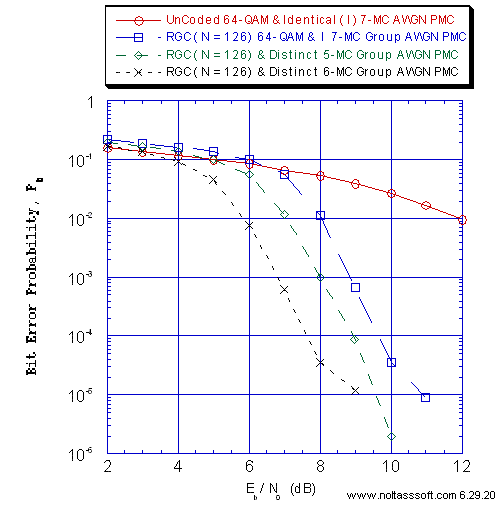```Figure 1. Bit Error Probability for UnCoded and (N = 126, j = 3, k = 6) Gallager
Coded Signaling over a Discrete-Time Waveform Additive White Gaussian Noise (AWGN)
Parallel MultiChannel (PMC) with AWGN:
```
```Equal probable Independent and Identical Distributed Source for 10,000,032 and
1,000,006 Information (Info) Bits for UnCoded and Regular Gallager Coded Signaling
respectively over a Discrete-Time Waveform (DTW) PMC Channel;
```
```N = 126, j = 3, k = 6, L = 65, Rate = 0.516 Regular Gallager Code (RGC) (T1 V2
Computer-generated);
```
```The I 7-MC G Signaling Schemes consist of {li] = {6,…, 6} <=> {64-QAM,…,64-QAM};
The D 5-MC G Signaling Schemes consist of {li} = {1,2,6,6,6} <=> {BPSK,QPSK,64-QAM,
64-QAM,64-QAM};
The D 6-MC G Signaling Schemes consist of {li} = {1,2,2,4,6,6} <=> {BPSK, QPSK,QPSK,
16-QAM,64-QAM,64-QAM};
```
```For each simulated Pb value, Eb/N0 = Eb/N0(1) = Eb/N0(2) = … = Eb/N0(K), for 1
through K Signaling Schemes;
```
```Each UnCoded or Gallager Coded DTW AWGN PMC subchannel consists of half-cosine
orthonormal baseband shaping pulse, 8 symbols per symbol period, and half-cosine
matched filter demodulator front-end;
```
```These DTW subchannels possess a NonDistorting, UnRestricted Bandwidth; &
```
```Sum-Product Algorithm Iterative Decoder using Model 2 (Check Messages then Bit
Messages Iteration Processing), Theoretical SPA Implementation Type, and
Maximum Number of Iterations per Block (Imax) = 50.
```
```Note: Zero Info Bit Error simulated for SNR = 10 dB (D 6-MC G); 11 & 12 dB
(D 5-MC G); & 12 dB (I 7-MC G).
```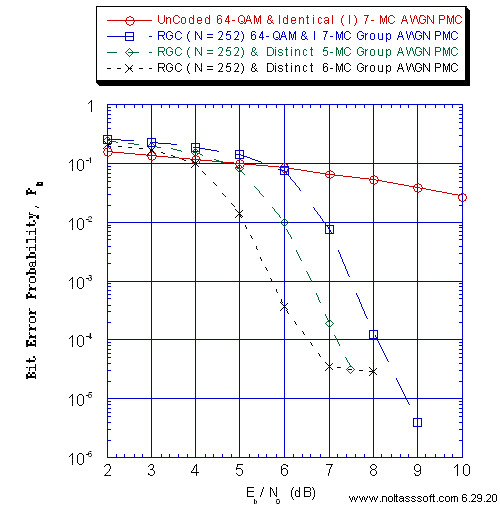```Figure 2. Bit Error Probability for UnCoded and (N = 252, j = 3, k = 4) Gallager
Coded Signaling over a Discrete-Time Waveform Additive White Gaussian Noise (AWGN)
Parallel MultiChannel (PMC) with AWGN:
```
```Equal probable Independent and Identical Distributed Source for 10,000,032 and
1,000,025 Information (Info) Bits for UnCoded and Regular Gallager Coded Signaling
respectively over a Discrete-Time Waveform (DTW) PMC Channel;
```
```N = 252, j = 3, k = 4, L = 65, Rate = 0.258 Regular Gallager Code (RGC) (T1 V2
Computer-generated);
```
```The I 7-MC G Signaling Schemes consist of {li] = {6,…,6} <=> {64-QAM,…,64-QAM};
The D 5-MC G Signaling Schemes consist of {li} = {1,2,6,6,6} <=> {BPSK,QPSK,64-QAM,
64-QAM,64-QAM};
The D 6-MC G Signaling Schemes consist of {li} = {1,2,2,4,6,6} <=> {BPSK,QPSK,QPSK,
16-QAM,64-QAM,64-QAM};
```
```For each simulated Pb value, Eb/N0 = Eb/N0(1) = Eb/N0(2) = … = Eb/N0(K), for 1
through K Signaling Schemes;
```
```Each UnCoded or Gallager Coded DTW AWGN PMC subchannel consists of half-cosine
orthonormal baseband shaping pulse, 8 symbols per symbol period, and half-cosine
matched filter demodulator front-end;
```
```These DTW subchannels possess a NonDistorting, UnRestricted Bandwidth; &
```
```Sum-Product Algorithm Iterative Decoder using Model 2 (Check Messages then Bit
Messages Iteration Processing), Theoretical SPA Implementation Type, and
Maximum Number of Iterations per Block (Imax) = 50.
```
```Note: Zero Info Bit Error simulated for SNR = 9 dB (D 6-MC G); 8, 9 & 10 dB
(D 5-MC G); & 10 dB (for I 7-MC G).
```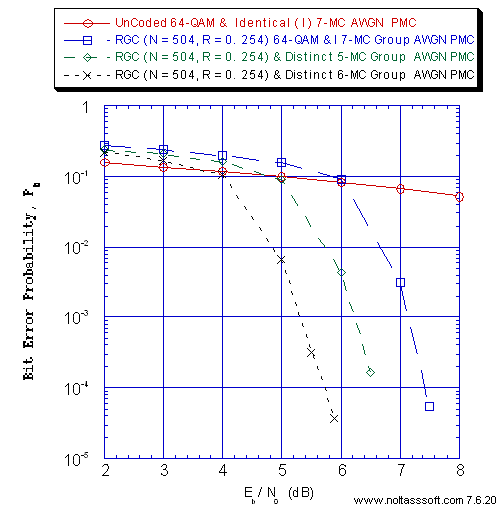```Figure 3. Bit Error Probability for UnCoded and (N = 504, j = 3, k = 4) Gallager
Coded Signaling over a Discrete-Time Waveform Additive White Gaussian Noise (AWGN)
Parallel MultiChannel (PMC) with AWGN:
```
```Equal probable Independent and Identical Distributed Source for 10,000,032 and
1,000,025 Information (Info) Bits for UnCoded and Regular Gallager Coded Signaling
respectively over a Discrete-Time Waveform (DTW) PMC Channel;
```
```N = 504, j = 3, k = 4, L = 128, Rate = 0.258 Regular Gallager Code (RGC) (T1 V2
Computer-generated);
```
```The I 7-MC G Signaling Schemes consist of {li] = {6,…,6 <=> {64-QAM,…,64-QAM};
The D 5-MC G Signaling Schemes consist of {li} = {1,2,6,6,6} <=> {BPSK,QPSK,64-QAM,
64-QAM,64-QAM};
The D 6-MC G Signaling Schemes consist of {li} = {1,2,2,4,6,6} <=> {BPSK,QPSK,QPSK,
16-QAM,64-QAM,64QAM};
```
```For each simulated Pb value, Eb/N0 = Eb/N0(1) = Eb/N0(2) = … = Eb/N0(K), for 1
through K Signaling Schemes;
```
```Each UnCoded or Gallager Coded DTW AWGN PMC subchannel consists of half-cosine
orthonormal baseband shaping pulse, 8 symbols per symbol period, and half-cosine
matched filter demodulator front-end;
```
```These DTW subchannels possess a NonDistorting, UnRestricted Bandwidth; &
```
```Sum-Product Algorithm Iterative Decoder using Model 2 (Check Messages then Bit
Messages Iteration Processing), Theoretical SPA Implementation Type, and
Maximum Number of Iterations per Block (Imax) = 50.
```
```Note Zero Info Bit Error simulated for SNR = 6 &7 dB (D 6-MC); 7, 7.5 & 8 dB
(D 5-MC); & 8 dB (I 7-MC G).
```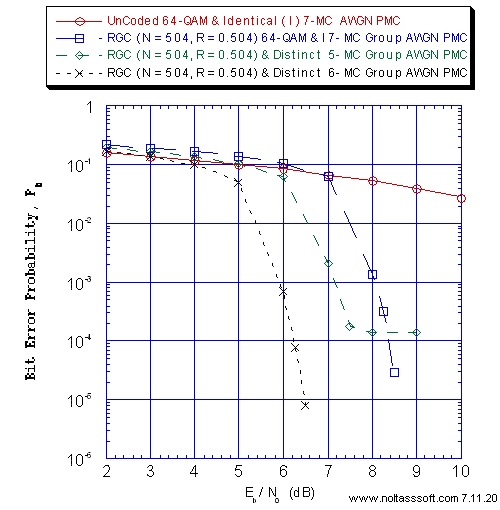```Figure 4.  Bit Error Probability for UnCoded and (N = 504, j = 3, k = 6) Gallager
Coded Signaling over a Discrete-Time Waveform Additive White Gaussian Noise
(AWGN) Parallel MultiChannel (PMC) with AWGN:
```
```Equal probable Independent and Identical Distributed Source for 10,000,032 and
1,000,252 Information (Info) Bits for UnCoded and Regular Gallager Coded Signaling
respectively over a Discrete-Time Waveform (DTW) PMC Channel;
```
```N = 504, j = 3, k = 6, L = 254, Rate = 0.504 Regular Gallager Code (RGC) (T1 V2
Computer-generated);
```
```The I 7-MC G Signaling Schemes consist of {li] = {6,…,6} <=> {64-QAM,…,64-QAM};
The D 5-MC G Signaling Schemes consist of {li} = {1,2,6,6,6} <=> {BPSK,QPSK,64-QAM,
64-QAM,64-QAM};
The D 6-MC G Signaling Schemes consist of {li} = {1,2,2,4,6,6} <=>{BPSK, QPSK,QPSK,
16-QAM,64-QAM,64-QAM};
```
```For each simulated Pb value, Eb/N0 = Eb/N0(1) = Eb/N0(2) = … = Eb/N0(K), for 1
through K Signaling Schemes;
```
```Each UnCoded or Gallager Coded DTW AWGN PMC subchannel consists of half-cosine
orthonormal baseband shaping pulse, 8 symbols per symbol period, and half-cosine
matched filter demodulator front-end;
```
```These DTW subchannels possess a NonDistorting, UnRestricted Bandwidth; &
```
```Sum-Product Algorithm Iterative Decoder using Model 2 (Check Messages then Bit
Messages Iteration Processing), Theoretical SPA Implementation Type, and
Maximum Number of Iterations per Block (Imax) = 50.
```
```Note: Zero Info Bits Error simulated for SNR = 7 dB (D 6-MC G); 10 dB (D 5-MC G);
& 9 & 10 dB (I 7-MC G);
```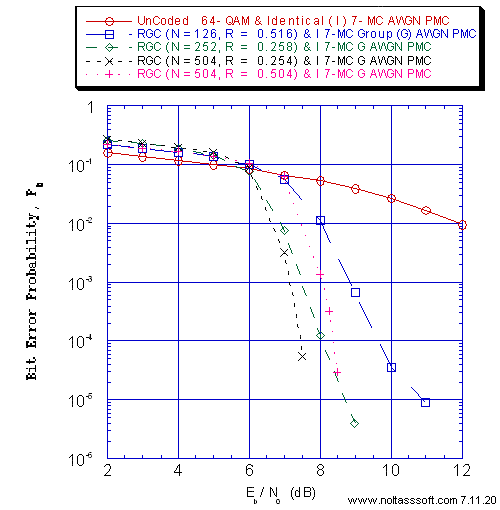```Figure 5. Bit Error Probability for UnCoded and Gallager Coded Signaling over a
Discrete-Time Waveform Additive White Gaussian Noise (AWGN) Parallel MultiChannel
(PMC) for 64-QAM Identical 7-MC Group with AWGN:
```
```Equal probable i.i.d. Source for 10,000,032, 1,000,025, 1,000,025, 1,000,064, and
1,000,252 Information (Info) Bits for N = 126; N = 252; N = 504, k = 4; N = 504,
k = 6 Regular Gallager Coded Signaling respectively over a Discrete-Time Waveform
(DTW) PMC Channel;
```
```N = 126, j = 3, k = 6, L = 65, Rate = 0.266 Regular Gallager Code (RGC) (T1 V2
Computer-generated);
N = 252, j = 3, k = 4, L = 65, Rate = 0.258 RGC (T1 V2 Computer-generated);
N = 504, j = 3, k = 4, L = 128, Rate = 0.254 RGC (T1 V2 Computer-generated);
N = 504, j = 3, k = 6, L = 254, Rate = 0.504 RGC (T1 V2 Computer-generated);
```
```The I 7-MC G Signaling Schemes consist of {li] = {6,…,6} <=> {64-QAM,…,64-QAM};
```
```For each simulated Pb value, Eb/N0 = Eb/N0(1) = Eb/N0(2) = … = Eb/N0(K), for 1
through K Signaling Schemes;
```
```Each UnCoded or Gallager Coded DTW AWGN PMC subchannel consists of half-cosine
orthonormal baseband shaping pulse, 8 symbols per symbol period, and half-cosine
matched filter demodulator front-end;
```
```These DTW subchannels possess a NonDistorting, UnRestricted Bandwidth; &
```
```Sum-Product Algorithm Iterative Decoder using Model 2 (Check Messages then Bit
Messages Iteration Processing), Theoretical SPA Implementation Type, and
Maximum Number of Iterations per Block (Imax) = 50.
```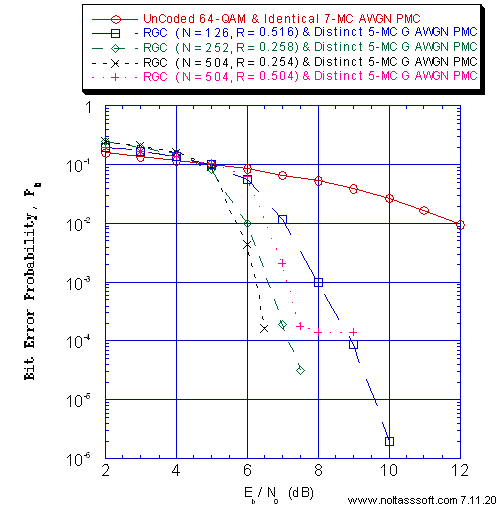```Figure 6. Bit Error Probability for UnCoded and Gallager Coded Signaling over a
Discrete-Time Waveform Additive White Gaussian Noise (AWGN) Parallel MultiChannel
(PMC) for Distinct 5-MC Group (G) with AWGN:
```
```Equal probable i.i.d. Source for 10,000,032, 1,000,025, 1,000,025, 1,000,064, and
1,000,252 Information (Info) Bits for N = 126; N = 252; N = 504, k = 4; N = 504,
k = 6 Regular Gallager Coded Signaling respectively over a Discrete-Time Waveform
(DTW) PMC Channel;
```
```N = 126, j = 3, k = 6, L = 65, Rate = 0.266 Regular Gallager Code (RGC) (T1 V2
Computer-generated);
N = 252, j = 3, k = 4, L = 65, Rate = 0.258 RGC (T1 V2 Computer-generated);
N = 504, j = 3, k = 4, L = 128, Rate = 0.254 RGC (T1 V2 Computer-generated);
N = 504, j = 3, k = 6, L = 254, Rate = 0.504 RGC (T1 V2 Computer-generated);
```
```The D 5-MC G Signaling Scheme consists of {li} = {1,2,6,6,6} <=> {BPSK,QPSK,64-QAM,
64-QAM,64-QAM};
```
```For each simulated Pb value, Eb/N0 = Eb/N0(1) = Eb/N0(2) = … = Eb/N0(K), for 1
through K Signaling Schemes;
```
```Each UnCoded or Gallager Coded DTW AWGN PMC subchannel consists of half-cosine
orthonormal baseband shaping pulse, 8 symbols per symbol period, and half-cosine
matched filter demodulator front-end;
```
```These DTW subchannels possess a NonDistorting, UnRestricted Bandwidth; &
```
```Sum-Product Algorithm Iterative Decoder using Model 2 (Check Messages then
Bit Messages Iteration Processing), Theoretical SPA Implementation Type, and
Maximum Number of Iterations per Block (Imax) = 50.
```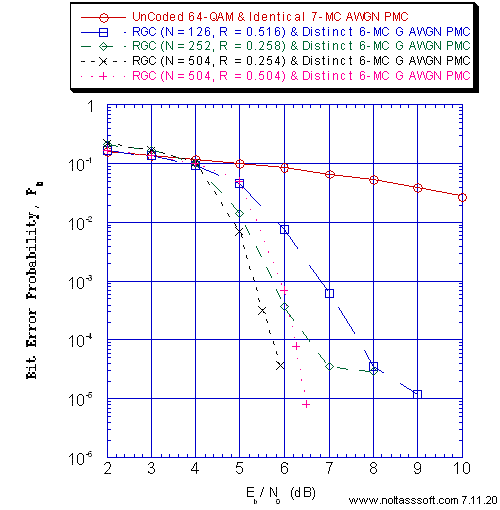```Figure 7. Bit Error Probability for UnCoded and Gallager Coded Signaling over a
Discrete-Time Waveform Additive White Gaussian Noise (AWGN) Parallel MultiChannel
(PMC) for Distinct 6-MC Group (G) with AWGN:
```
```Equal probable i.i.d. Source for 10,000,032, 1,000,025, 1,000,025, 1,000,064, and
1,000,252 Information (Info) Bits for N = 126; N = 252; N = 504, k = 4; N = 504,
k = 6 Regular Gallager Coded Signaling respectively over a Discrete-Time
Waveform (DTW) PMC Channel;
```
```N = 126, j = 3, k = 6, L = 65, Rate = 0.266 Regular Gallager Code (RGC) (T1 V2
Computer-generated);
N = 252, j = 3, k = 4, L = 65, Rate = 0.258 RGC (T1 V2 Computer-generated);
N = 504, j = 3, k = 4, L = 128, Rate = 0.254 RGC (T1 V2 Computer-generated);
N = 504, j = 3, k = 6, L = 254, Rate = 0.504 RGC (T1 V2 Computer-generated);
```
```The D 6-MC G Signaling Scheme consists of {li} = {1,2,2,4,6,6} <=> {BPSK,QPSK,QPSK,
16-QAM,64-QAM,64-QAM};
```
```For each simulated Pb value, Eb/N0= Eb/N0(1) = Eb/N0(2) = … = Eb/N0(K), for 1
through K Signaling Schemes;
```
```Each UnCoded or Gallager Coded DTW AWGN PMC subchannel consists of half-cosine
orthonormal baseband shaping pulse, 8 symbols per symbol period, and half-cosine
matched filter demodulator front-end;
```
```These DTW subchannels possess a NonDistorting, UnRestricted Bandwidth; &
```
```Sum-Product Algorithm Iterative Decoder using Model 2 (Check Messages then Bit
Messages Iteration Processing), Theoretical SPA Implementation Type, and Maximum
Number of Iterations per Block (Imax) = 50.
```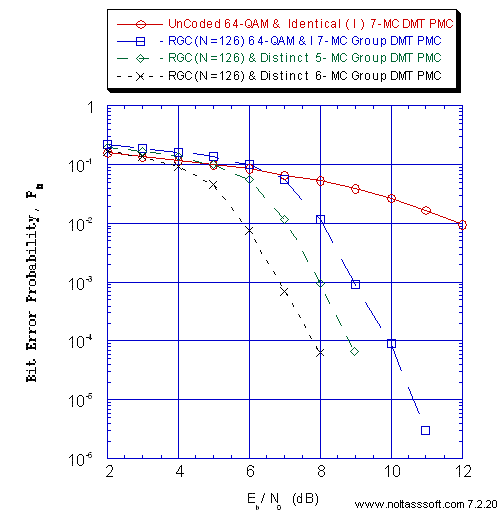```Figure 8. Bit Error Probability for UnCoded and (N = 126, j = 3, k = 6) Gallager
Coded Signaling over a Discrete-Time Discrete MultiTone (DMT) Modulation Parallel
MultiCarrier/MultiChannel (PMC) with Additive White Gaussian Noise (AWGN):
```
```Equal probable Independent and Identical Distributed Source for 10,000,032 and
1,000,006 Information (Info) Bits for UnCoded and Regular Gallager Coded Signaling
respectively over a Discrete-Time (DT) DMT PMC Channel;
```
```N = 126, j = 3, k = 6, L = 65, Rate = 0.516 Regular Gallager Code (RGC) (T1 V2
Computer-generated);
```
```The I 7-MC G Signaling Schemes consists of {li] = {6,…,6} <=> {64-QAM,…,64-QAM};
The D 5-MC G Signaling Scheme consists of {li} = {1,2,6,6,6} <=> {BPSK,QPSK,64-QAM,
64-QAM,64-QAM};
The D 6-MC G Signaling Scheme consists of {li} = {1,2,2,4,6,6} <=> {BPSK, QPSK,QPSK,
16-QAM,64-QAM,64-QAM};
```
```For each simulated Pb value, Eb/N0 = Eb/N0(1) = Eb/N0(2) = … = Eb/N0(K), for 1
through K Signaling Schemes;
```
```The number of IDFT Samples for the MultiCarrier Signal DT Waveform for UC Identical
(I) 7-MC; RGC I 7-MC G, Distinct 5-MC G, Distinct 6-MC G cases are 16, 64, 64, and
128, respectively;
```
```These DT DMT PMC channels possess a NonDistorting, UnRestricted Bandwidth; &
```
```Sum-Product Algorithm Iterative Decoder using Model 2 (Check Messages then Bit
Messages Iteration Processing), Theoretical SPA Implementation Type, and
Maximum Number of Iterations per Block (Imax) = 50.
```
```Note: Zero Info Bit Error simulated for SNR = 9 &10 dB (D 6-MC G); 10, 11 & 12 dB
(D 5-MC G); & 12 dB (I 7-MC G).
```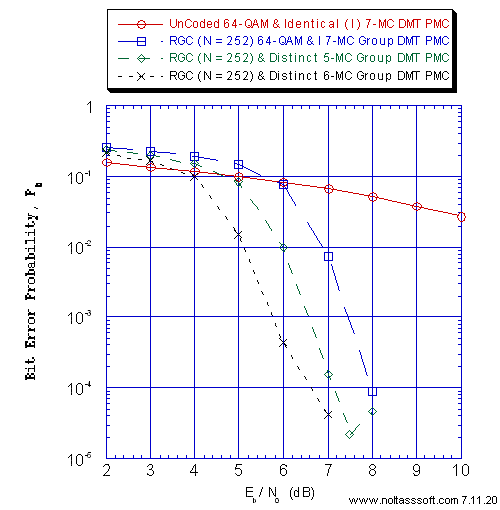```Figure 9. Bit Error Probability for UnCoded and (N = 252, j = 3, k = 4) Gallager
Coded Signaling over a Discrete-Time Discrete MultiTone (DMT)  Modulation Parallel
MultiCarrier/MultiChannel (PMC) with Additive White Gaussian Noise (AWGN):
```
```Equal probable Independent and Identical Distributed Source for 10,000,032 and
1,000,025 Information Bits for UnCoded and Regular Gallager Coded Signaling
respectively over a Discrete-Time (DT) DMT PMC Channel;
```
```N = 252, j = 3, k = 4, L = 65, Rate = 0.258 Regular Gallager Code (RGC) (T1 V2
Computer-generated);
```
```The I 7-MC G Signaling Schemes consists of {li] = {6,…,6 <=> {64-QAM,…,64-QAM};
The D 5-MC G Signaling Scheme consists of {li} = {1,2,6,6,6} <=> {BPSK,QPSK,64-QAM,
64-QAM,64-QAM};
The D 6-MC G Signaling Scheme consists of {li} = {1,2,2,4,6,6} <=> {BPSK,QPSK,QPSK,
16-QAM,64-QAM,64-QAM};
```
```For each simulated Pb value, Eb/N0 = Eb/N0(1) = Eb/N0(2) = … = Eb/N0(K) , for 1
through K Signaling Schemes;
```
```The number of IDFT Samples for the MultiCarrier Signal DT Waveform for UC Identical
(I) 7-MC; RGC I 7-MC G, Distinct 5-MC G, Distinct 6-MC G cases are 16, 128, 128,
and 256, respectively;
```
```These DT DMT PMC channels possess a NonDistorting, UnRestricted Bandwidth; &
```
```Sum-Product Algorithm Iterative Decoder using Model 2 (Check Messages then Bit
Messages Iteration Processing), Theoretical SPA Implementation Type, and
Maximum Number of Iterations per Block (Imax) = 50.
```
```Note: Zero Info Bit Error occurred for SNR = 8 & 9 dB (D 6-MC); 9 & 10
(D 5-MC G); & 9 & 10 dB (I 7-MC G).
```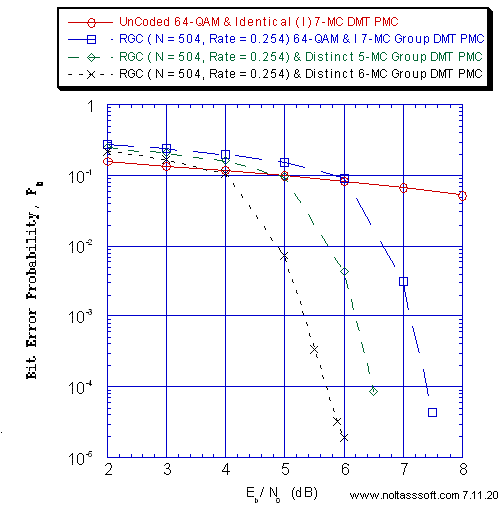```Figure 10. Bit Error Probability for UnCoded and (N = 504, j = 3, k = 4) Gallager
Coded Signaling over a Discrete-Time Discrete MultiTone (DMT)  Modulation
Parallel MultiCarrier/MultiChannel (PMC) with Additive White Gaussian Noise (AWGN):
```
```Equal probable Independent and Identical Distributed Source for 10,000,032 and
1,000,064 Information Bits for UnCoded and Regular Gallager Coded Signaling
respectively over a Discrete-Time (DT) DMT PMC Channel;
```
```N = 504, j = 3, k = 4, L = 128, Rate = 0.254 Regular Gallager Code (RGC) (T1 V2
Computer-generated);
```
```The I 7-MC G Signaling Schemes consists of {li] = {6,…,6} <=> {64-QAM,…,64-QAM};
The D 5-MC G Signaling Scheme consists of {li} = {1,2,6,6,6} <=> {BPSK,QPSK,64-QAM,
64-QAM,64-QAM};
The D 6-MC G Signaling Scheme consists of {li} = {1,2,2,4,6,6} <=> {BPSK, QPSK,QPSK,
64-QAM,64-QAM};
```
```For each simulated Pb value, Eb/N0 = Eb/N0(1) = Eb/N0(2) = … = Eb/N0(K), for 1
through K Signaling Schemes;
```
```The number of IDFT Samples for the MultiCarrier Signal DT Waveform for UC Identical
(I) 7-MC; RGC I 7-MC G, Distinct 5-MC G, Distinct 6-MC G cases are 16, 256, 256,
and 512, respectively;
```
```These DT DMT PMC channels possess a NonDistorting, UnRestricted Bandwidth; &
```
```Sum-Product Algorithm Iterative Decoder using Model 2 (Check Messages then Bit
Messages Iteration Processing), Theoretical SPA Implementation Type, and
Maximum Number of Iterations per Block (Imax) = 50.
```
```Note: Zero Info Bit Error simulated for SNR = 7 dB (D 6-MC G); 7, 7.5, & 8 dB
(D 5-MC G); & 8 dB (I 7-MC G);
```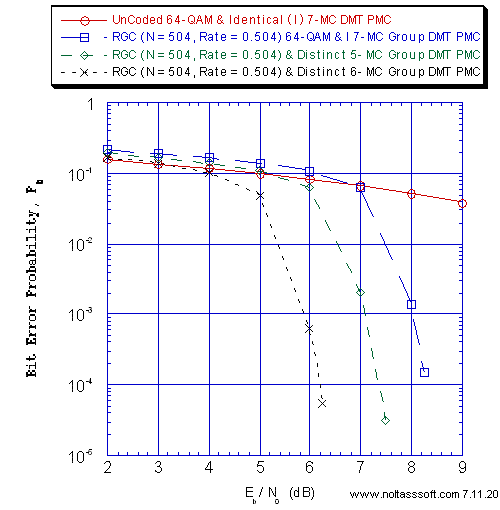```Figure 11. Bit Error Probability for UnCoded and (N = 504, j = 3, k = 6) Gallager
Coded Signaling over a Discrete-Time Discrete MultiTone (DMT)  Modulation Parallel
MultiCarrier/MultiChannel (PMC) with Additive White Gaussian Noise (AWGN):
```
```Equal probable Independent and Identical Distributed Source for 10,000,032 and
1,000,252 Information Bits for UnCoded and Regular Gallager Coded Signaling
respectively over a Discrete-Time (DT) DMT PMC Channel;
```
```N = 504, j = 3, k = 6, L = 254, Rate = 0.504 Regular Gallager Code (RGC) (T1 V2
Computer-generated);
```
```The I 7-MC G Signaling Schemes consists of {li] = {6,…,6} <=> {64-QAM,…,64-QAM};
The D 5-MC G Signaling Scheme consists of {li} = {1,2,6,6,6} <=> {BPSK,QPSK,64-QAM,
64-QAM,64-QAM};
The D 6-MC G Signaling Scheme consists of {li} = {1,2,2,4,6,6} <=> {BPSK, QPSK,QPSK,
16-QAM,64-QAM,64-QAM};
```
```For each simulated Pb value, Eb/N0 = Eb/N0(1) = Eb/N0(2) = … = Eb/N0(K) , for 1
through K Signaling Schemes;
```
```The number of IDFT Samples for the MultiCarrier Signal DT Waveform for UC Identical
(I) 7-MC; RGC I 7-MC G, Distinct 5-MC G, Distinct 6-MC G cases are 16, 256, 256,
and 512, respectively;
```
```These DT DMT PMC channels possess a NonDistorting, UnRestricted Bandwidth; &
```
```Sum-Product Algorithm Iterative Decoder using Model 2 (Check Messages then Bit
Messages Iteration Processing), Theoretical SPA Implementation Type, and
Maximum Number of Iterations per Block (Imax) = 50.
```
```Note: Zero Info Bit Error simulated for SNR = 6.5 & 7 dB (D 6-MC G); 8, 9 &10 dB
(D 5-MC G); 8.5, 9, & 10 dB (I 7-MC G);
```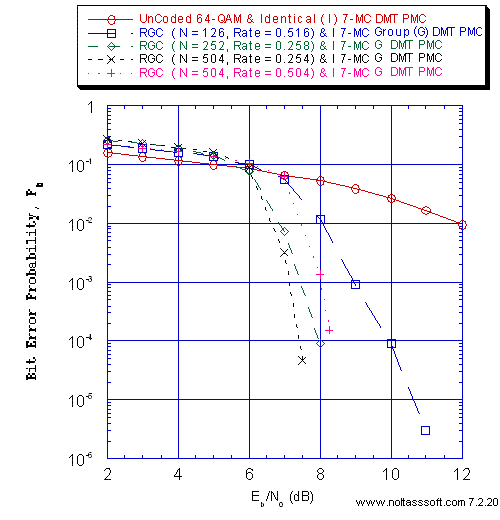```Figure 12. Bit Error Probability for UnCoded and Gallager Coded Signaling over a
Discrete-Time Discrete MultiTone (DMT) Parallel MultiCarrier/MultiChannel
(PMC) for 64-QAM Identical 7-MC Group with Additive White Gaussian Noise (AWGN):
```
```Equal probable i.i.d. Source for 10,000,032, 1,000,025, 1,000,025, 1,000,064, and
1,000,252 Information (Info) Bits for N = 126; N = 252; N = 504, k = 4; N = 504,
k = 6 Regular Gallager Coded Signaling respectively over a Discrete-Time (DT) DMT
PMC Channel;
```
```N = 126, j = 3, k = 6, L = 65, Rate = 0.266 Regular Gallager Code (RGC) (T1 V2
Computer-generated);
N = 252, j = 3, k = 4, L = 65, Rate = 0.258 RGC (T1 V2 Computer-generated);
N = 504, j = 3, k = 4, L = 128, Rate = 0.254 RGC (T1 V2 Computer-generated);
N = 504, j = 3, k = 6, L = 254, Rate = 0.504 RGC (T1 V2 Computer-generated);
```
```The I 7-MC G Signaling Schemes consists of {li] = {6,…,6} <=> {64-QAM,…,64-QAM};
```
```For each simulated Pb value, Eb/N0 = Eb/N0(1) = Eb/N0(2) = … = Eb/N0(K) , for 1
through K Signaling Schemes;
```
```These DT DMT PMC channels possess a NonDistorting, UnRestricted Bandwidth; &
```
```Sum-Product Algorithm Iterative Decoder using Model 2 (Check Messages then Bit
Messages Iteration Processing), Theoretical SPA Implementation Type, and
Maximum Number of Iterations per Block (Imax) = 50.
```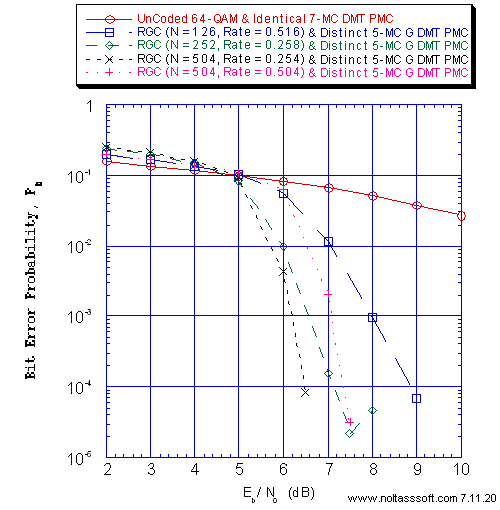```Figure 13. Bit Error Probability for UnCoded and Gallager Coded Signaling over a
Discrete-Time Discrete MultiTone (DMT) Modulation Parallel MultiCarrier/MultiChannel
(PMC) for Distinct 5-MC Group (G) with Additive White Gaussian Noise (AWGN):
```
```Equal probable i.i.d. Source for 10,000,032, 1,000,025, 1,000,025, 1,000,064, and
1,000,252 Information (Info) Bits for N = 126; N = 252; N = 504, k = 4; N = 504,
k = 6 Regular Gallager Coded Signaling respectively over a Discrete-Time (DT)
DMT PMC Channel;
```
```N = 126, j = 3, k = 6, L = 65, Rate = 0.266 Regular Gallager Code (RGC) (T1 V2
Computer-generated);
N = 252, j = 3, k = 4, L = 65, Rate = 0.258 RGC (T1 V2 Computer-generated);
N = 504, j = 3, k = 4, L = 128, Rate = 0.254 RGC (T1 V2 Computer-generated);
N = 504, j = 3, k = 6, L = 254, Rate = 0.504 RGC (T1 V2 Computer-generated);
```
```The D 5-MC G Signaling Scheme consists of {li} = {1,2,6,6,6} <=> {BPSK,QPSK,64-QAM,
64-QAM,64-QAM};
```
```For each simulated Pb value, Eb/N0 = Eb/N0(1) = Eb/N0(2) = … = Eb/N0(K) , for 1
through K Signaling Schemes;
```
```These DT DMT PMC channels possess a NonDistorting, UnRestricted Bandwidth; &
```
```Sum-Product Algorithm Iterative Decoder using Model 2 (Check Messages then Bit
Messages Iteration Processing), Theoretical SPA Implementation Type, and
Maximum Number of Iterations per Block (Imax) = 50.
```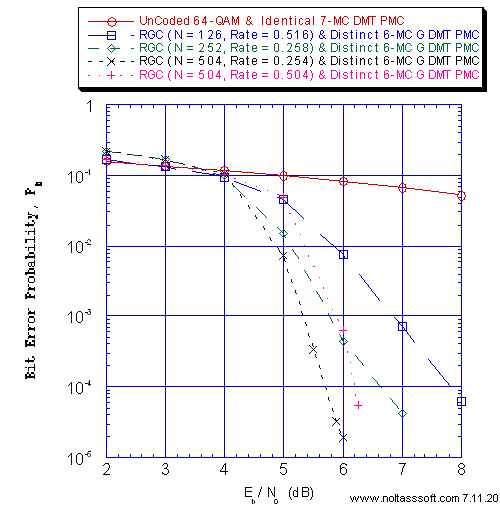```Figure 14. Bit Error Probability for UnCoded and Gallager Coded Signaling over a
Discrete-Time Discrete MultiTone (DMT) Modulation Parallel MultiCarrier/MultiChannel
(PMC) for Distinct 6-MC Group (G) with Additive White Gaussian Noise (AWGN):
```
```Equal probable i.i.d. Source for 10,000,032, 1,000,025, 1,000,025, 1,000,064, and
1,000,252 Information (Info) Bits for N = 126; N = 252; N = 504, k = 4; N = 504,
k = 6 Regular Gallager Coded Signaling respectively over a Discrete-Time (DT) DMT
PMC Channel;
```
```N = 126, j = 3, k = 6, L = 65, Rate = 0.266 Regular Gallager Code (RGC) (T1 V2
Computer-generated);
N = 252, j = 3, k = 4, L = 65, Rate = 0.258 RGC (T1 V2 Computer-generated);
N = 504, j = 3, k = 4, L = 128, Rate = 0.254 RGC (T1 V2 Computer-generated);
N = 504, j = 3, k = 6, L = 254, Rate = 0.504 RGC (T1 V2 Computer-generated);
```
```The D 6-MC G Signaling Schemes consist of {li} = {1,2,2,4,6,6} <=> {BPSK, QPSK,QPSK,
16-QAM,64-QAM,64-QAM};
```
```For each simulated Pb value, Eb/N0 = Eb/N0(1) = Eb/N0(2) = … = Eb/N0(K) , for 1
through K Signaling Schemes;
```
```These DT DMT PMC channels possess a NonDistorting, UnRestricted Bandwidth; &
```
```Sum-Product Algorithm Iterative Decoder using Model 2 (Check Messages then Bit
Messages Iteration Processing), Theoretical SPA Implementation Type, and
Maximum Number of Iterations per Block (Imax) = 50.
```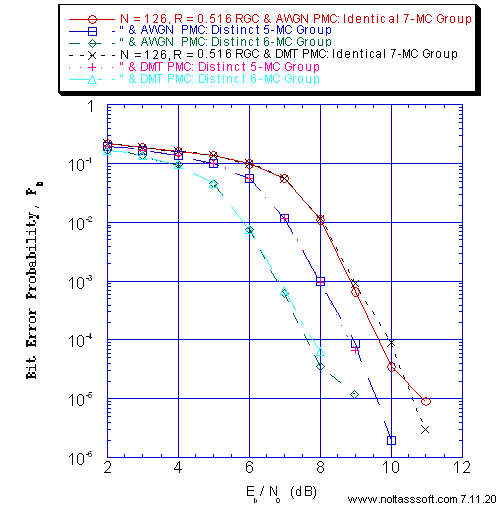```Figure 15. Bit Error Probability for (N = 126, j = 3, k = 6) Gallager Coded
Signaling over a Discrete-Time Waveform Additive White Gaussian Noise (AWGN)
Parallel MultiChannel (PMC) with AWGN & Discrete-Time Discrete MultiTone (DMT)
Modulation Parallel MultiCarrier/MultiChannel (PMC) with AWGN:
```
```Equal probable Independent and Identical Distributed Source for 1,000,025
Information Bits for Regular Gallager Coded Signaling over a Discrete-Time
Waveform (DTW) AWGN PMC and Discrete-Time (DT) DMT PMC Channel;
```
```N = 126, j = 3, k = 6, L = 65, Rate = 0.516 Regular Gallager Code (T1 V2
Computer-generated);
```
```The I 7-MC Group (G) Signaling Schemes consist of {li] = {6,…,6} <=> {64-QAM,…,
64-QAM};
The D 5-MC G Signaling Scheme consist of {li} = {1,2,6,6,6} <=> {BPSK,QPSK,64-QAM,
64-QAM,64-QAM};
The D 6-MC G Signaling Scheme consist of {li} = {1,2,2,4,6,6} <=> {BPSK, QPSK,QPSK,
64-QAM,64-QAM};
```
```For each simulated Pb value, Eb/N0 = Eb/N0(1) = Eb/N0(2) = … = Eb/N0(K) , for 1
through K Signaling Schemes;
```
```These DTW subchannels and DT DMT PMC channels possess a NonDistorting,
UnRestricted Bandwidth; &
```
```Sum-Product Algorithm Iterative Decoder using Model 2 (Check Messages then Bit
Messages Iteration Processing), Theoretical SPA Implementation Type, and
Maximum Number of Iterations per Block (Imax) = 50.
```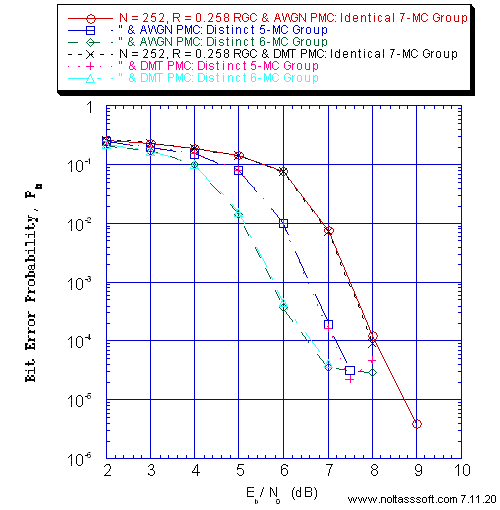```Figure 16. Bit Error Probability for (N = 252, j = 3, k = 4) Gallager Coded
Signaling over a Discrete-Time Waveform Additive White Gaussian Noise (AWGN)
Parallel MultiChannel (PMC) with AWGN & Discrete-Time Discrete MultiTone (DMT)
Modulation Parallel MultiCarrier/MultiChannel (PMC) with AWGN:
```
```Equal probable Independent and Identical Distributed Source for 1,000,025
Information Bits for Regular Gallager Coded Signaling over a Discrete-Time
Waveform (DTW) AWGN PMC and Discrete-Time (DT) DMT PMC Channel;
```
```N = 252, j = 3, k = 4, L = 65, Rate = 0.258 Regular Gallager Code (T1 V2
Computer-generated);
```
```The I 7-MC Group (G) Signaling Schemes consist of {li] = {6,…,6} <=> {64-QAM,…,
64-QAM};
The D 5-MC G Signaling Scheme consist of {li} = {1,2,6,6,6} <=> {BPSK,QPSK,64-QAM,
64-QAM,64-QAM};
The D 6-MC G Signaling Scheme consist of {li} = {1,2,2,4,6,6} <=> {BPSK, QPSK,QPSK,
64-QAM,64-QAM};
```
```For each simulated Pb value, Eb/N0 = Eb/N0(1) = Eb/N0(2) = … = Eb/N0(K), for 1
through K Signaling Schemes;
```
```These DTW subchannels and DT DMT PMC channels possess a NonDistorting,
UnRestricted Bandwidth; &
```
```Sum-Product Algorithm Iterative Decoder using Model 2 (Check Messages then Bit
Messages Iteration Processing), Theoretical SPA Implementation Type, and
Maximum Number of Iterations per Block (Imax) = 50.
```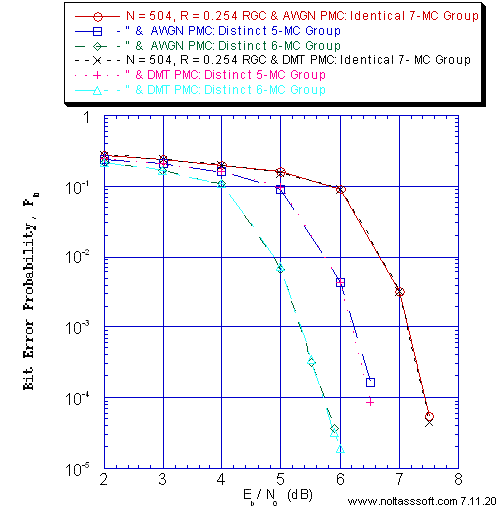```Figure 17. Bit Error Probability for (N = 504, j = 3, k = 4) Gallager Coded
Signaling over a Discrete-Time Waveform Additive White Gaussian Noise (AWGN)
Parallel MultiChannel (PMC) with AWGN & Discrete-Time Discrete MultiTone (DMT)
Modulation Parallel MultiCarrier/MultiChannel (PMC) with AWGN:
```
```Equal probable Independent and Identical Distributed Source for 1,000,064
Information Bits for Regular Gallager Coded Signaling over a Discrete-Time
Waveform (DTW) AWGN PMC and Discrete-Time (DT) DMT PMC Channel;
```
```N = 504, j = 3, k = 4, L = 128, Rate = 0.254 Regular Gallager Code (T1 V2
Computer-generated);
```
```The I 7-MC Group (G) Signaling Schemes consist of {li] = {6,…,6} <=> {64-QAM,…,
64-QAM};
The D 5-MC G Signaling Scheme consist of {li} = {1,2,6,6,6} <=> {BPSK,QPSK,64-QAM,
64-QAM,64-QAM};
The D 6-MC G Signaling Scheme consist of {li} = {1,2,2,4,6,6} <=> {BPSK, QPSK,QPSK,
64-QAM,64-QAM};
```
```For each simulated Pb value, Eb/N0 = Eb/N0(1) = Eb/N0(2) = … = Eb/N0(K) , for 1
through K Signaling Schemes;
```
```These DTW subchannels and DT DMT PMC channels possess a NonDistorting,
UnRestricted Bandwidth; &
```
```Sum-Product Algorithm Iterative Decoder using Model 2 (Check Messages then Bit
Messages Iteration Processing), Theoretical SPA Implementation Type, and
Maximum Number of Iterations per Block (Imax) = 50.
```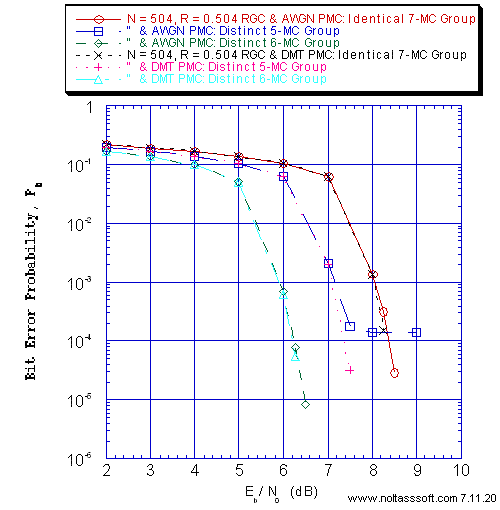```Figure 18. Bit Error Probability for (N = 504, j = 3, k = 6) Gallager Coded
Signaling over a Discrete-Time Waveform Additive White Gaussian Noise (AWGN)
Parallel MultiChannel (PMC) with AWGN & Discrete-Time Discrete MultiTone (DMT)
Modulation Parallel MultiCarrier/MultiChannel (PMC) with AWGN:
```
```Equal probable Independent and Identical Distributed Source for 1,000,252
Information Bits for Regular Gallager Coded Signaling over a Discrete-Time
Waveform (DTW) AWGN PMC and Discrete-Time (DT) DMT PMC Channel;
```
```N = 504, j = 3, k = 6, L = 254, Rate = 0.504 Regular Gallager Code (T1 V2
Computer-generated);
```
```The I 7-MC Group (G) Signaling Schemes consist of {li] = {6,…,6} <=> {64-QAM,…,
64-QAM};
The D 5-MC G Signaling Scheme consist of {li} = {1,2,6,6,6} <=> {BPSK,QPSK,64-QAM,
64-QAM,64-QAM};
The D 6-MC G Signaling Scheme consist of {li} = {1,2,2,4,6,6} <=> {BPSK,QPSK,QPSK,
64-QAM,64-QAM};
```
```For each simulated Pb value, Eb/N0 = Eb/N0(1) = Eb/N0(2) = … = Eb/N0(K) , for 1
through K Signaling Schemes;
```
```These DTW subchannels and DT DMT PMC channels possess a NonDistorting,
UnRestricted Bandwidth; &
```
```Sum-Product Algorithm Iterative Decoder using Model 2 (Check Messages then Bit
Messages Iteration Processing), Theoretical SPA Implementation Type, and
Maximum Number of Iterations per Block (Imax) = 50.
```

```BUY T1 Version 2 (ADVDCSMT1DCSS Professional software system tool)NOW.
```